## Demo

D.J.Peters
Posts: 8023
Joined: May 28, 2005 3:28
Contact:

### Demo

Code: Select all

`const g         as single  = 9.81const g2        as single  = g * 2.0const gd2       as single  = g * 0.5const pi        as single  = atn(1)*4.0const pi2       as single  = pi * 2.0const onedegree as single  = pi/180.0const max_particles as integer = 5000const last_particle as integer = max_particles - 1type PARTICLE  bt   as double  scrx as integer  scry as integer  posx as integer  posy as integer  v0   as single  a    as single  col  as ulongend typedim shared particles(last_particle) as PARTICLEsub init_particle(byval i as integer,byval t as double)  static w as single  dim as single rc,gc,bc  rc=cos(w)*0.5+0.5  gc=cos(w*1.25)*0.5+0.5  bc=cos(w*1.5)*0.5+0.5  with particles(i)    .posx=128+int(cos(w)*128)    .posy=128+int(sin(w)*128)    .bt = t    .v0 = sin(w)*80    .a  = cos(w)*pi+pi2*rnd    .col=rgb(255*rc,255*gc,255*bc)  end with  w=w+(1.0/max_particles)end subsub update_particles(byval t as double)  dim as single s,vs  for i as integer=0 to last_particle    with particles(i)    s=(t - .bt):vs=.v0*s    .scrx=128 +.posx + int(vs*cos(.a))    .scry=128 -(.posy + int(vs*sin(.a)-gd2*(s*s)))    end with  nextend subsub render_particles(byval p as ulong ptr,byval t as double)  static as single w=0 : w+=0.01  dim as ubyte c = (2-sin(w))*32  line p,(0,0)-step(255,255),0,BF  draw string p,(0,abs(sin(w))*240),"-- FreeBASIC --",&HFFFFFF  for i as integer = 0 to last_particle    with particles(i)      .scrx and=255      .scry and=255       pset p,(.scrx,.scry),.col    end with    if int(rnd*c)=64-c then init_particle(i,t)      nextend sub'' main'dim as integer w=640,h=480'screeninfo w,hscreenres w,h,32,,1setMouse  ,,0dim as integer TexturePitch,BufferPitch,TablePitchdim as ulong ptr pTexture,pBuffer,pTabledim as any ptr imgBuffer =imagecreate(w,h,0)dim as any ptr imgTable  =imagecreate(w,h,0)dim as any ptr imgTexture=imagecreate(256,256,0)imageinfo imgBuffer ,,,,BufferPitch ,pBufferimageinfo imgTable  ,,,,TablePitch  ,pTableimageinfo imgTexture,,,,TexturePitch,pTextureTexturePitch shr=2BufferPitch  shr=2TablePitch   shr=2for j as integer=0 to h-1  dim as single sy =  1 - j*(2/h)  for i as integer=0 to w-1    dim as single sx = -1 + i*(2/w)    dim as single sr = sqr(sx*sx+sy*sy)    dim as single sa = atan2(sy,sx)    dim as single su = 1/sr    dim as single sv = sa * 3/3.14159    dim as single sw = sr * sr    if ( sw>1 ) then sw=1    dim as long iu = su*255    dim as long iv = sv*255    dim as long iw = sw*255    pTable[j*TablePitch+i] = ((iw and &HFF) shl 16) or ((iv and &HFF) shl 8) or (iu and &HFF)  nextnext' maindim as double t=Timer()for index as integer=0 to last_particle  init_particle index,tnextdim as long framewhile inkey()=""  t=Timer()  update_particles t  render_particles imgTexture,t  frame+=5  for j as integer = 0 to h-1    for i as integer = 0 to w-1      dim as const long ival = pTable[j*TablePitch+i]      dim as const long icol = pTexture[ ( (ival and &H0000FFFF) + frame) and &H0000FFFF ]      dim as const long isca = ival shr 16      pBuffer[j*BufferPitch+i] = ((((icol and &H00FF00FF)*isca) shr 8) and &H00FF00FF) _                               + ((((icol and &H0000FF00)*isca) shr 8) and &H0000FF00)    next  next   put (0,0),imgBuffer,PSET  sleep 5wend`
BasicCoder2
Posts: 3525
Joined: Jan 01, 2009 7:03

### Re: Demo

And it runs on my old FreeBASIC Compiler - Version 0.24.0 :)
All it needs is music?
I wonder if it is possible to generate sound patterns as it is to generate visual patterns?
D.J.Peters
Posts: 8023
Joined: May 28, 2005 3:28
Contact:

### Re: Demo

Code: Select all

`const g         as single  = 9.80665const g2        as single  = g * 2.0const gd2       as single  = g * 0.5const pi        as single  = 3.141592654const pi2       as single  = pi * 2.0const onedegree as single  = pi/180.0const max_particles as integer = 10000const last_particle as integer = max_particles - 1type PARTICLE  bt  as double 'birth time   scrx as integer  scry as integer  posx as integer  posy as integer  v0  as single 'speed    at time 0  a   as single  col as integerend typedim shared particles(max_particles) as PARTICLEsub init_particle(byval i as integer,byval t as double)  static as single w,w2  dim as single rc,gc,bc,addi  rc=cos(w2     )*0.5+0.5  gc=cos(w2*1.25)*0.5+0.5  bc=cos(w2*1.5 )*0.5+0.5  addi=0.00001 + sin(w2)*0.000005  with particles(i)    .posx=int(cos(w*pi)*320)    .posy=int(sin(w)*240)    .bt = t    .v0 = sin(w)*80+41    .a  = cos(w*w)*pi +rnd*.5    .col=rgb(255*rc,255*gc,255*bc)  end with  w+ =addi  w2+=0.0001end subsub update_particles(byval t as double)  dim as single s,vs  dim i as integer  for i=0 to last_particle    with particles(i)    s=(t - .bt):vs=.v0*s    .scrx=.posx + int(vs*cos(.a))    .scry=.posy + int(vs*sin(.a)-gd2*(s*s))    end with  next end sub   sub render_particles(byval p as ulong ptr,byval t as double)  static as single w=0 : w+=0.01  line p,(0,0)-step(255,255),0,BF  for i as integer = 0 to last_particle    with particles(i)      .scrx and=255      .scry and=255       pset p,(.scrx,.scry),.col    end with    if int(rnd*100)=50 then init_particle(i,t)   next  draw string p,(0,abs(sin(w))*240),"-- FreeBASIC --",RGB(255,255,255)  end sub'' main'dim as integer w=640,h=480'screeninfo w,hscreenres w,h,32,,1setMouse  ,,0dim as integer TexturePitch,BufferPitch,TablePitchdim as ulong ptr pTexture,pBuffer,pTabledim as any ptr imgBuffer =imagecreate(w,h,0)dim as any ptr imgTable  =imagecreate(w,h,0)dim as any ptr imgTexture=imagecreate(256,256,0)imageinfo imgBuffer ,,,,BufferPitch ,pBufferimageinfo imgTable  ,,,,TablePitch  ,pTableimageinfo imgTexture,,,,TexturePitch,pTextureTexturePitch shr=2BufferPitch  shr=2TablePitch   shr=2for j as integer=0 to h-1  dim as single sy =  1 - j*(2/h)  for i as integer=0 to w-1    dim as single sx = -1 + i*(2/w)    dim as single sr = sqr(sx*sx+sy*sy)    dim as single sa = atan2(sy,sx)    dim as single su = 1/sr    dim as single sv = sa * 3/3.14159    dim as single sw = sr * sr    if ( sw>1 ) then sw=1    dim as long iu = su*255    dim as long iv = sv*255    dim as long iw = sw*255    pTable[j*TablePitch+i] = ((iw and &HFF) shl 16) or ((iv and &HFF) shl 8) or (iu and &HFF)  nextnext' maindim as double t=Timer()for index as integer=0 to last_particle  init_particle index,tnextdim as long framewhile inkey()=""  t=Timer()  update_particles t  render_particles imgTexture,t  frame+=8  for j as integer = 0 to h-1    for i as integer = 0 to w-1      dim as const long ival = pTable[j*TablePitch+i]      dim as const long icol = pTexture[ ( (ival and &H0000FFFF) + frame) and &H0000FFFF ]      dim as const long isca = ival shr 16      pBuffer[j*BufferPitch+i] = ((((icol and &H00FF00FF)*isca) shr 8) and &H00FF00FF) _                               + ((((icol and &H0000FF00)*isca) shr 8) and &H0000FF00)    next  next   put (0,0),imgBuffer,PSET  sleep 10wend`
Tyr_Anassazi
Posts: 28
Joined: Jul 01, 2013 15:01
Location: Russia, Novosibirsk
Contact:

### Re: Demo

Fantastic work!
Something like a black hole or wormhole.D.J.Peters
Posts: 8023
Joined: May 28, 2005 3:28
Contact:

### Re: Demo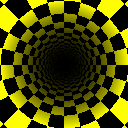Here are the description how it works.

The important part is the image in "imgTable" with the same resolution as the screen or window.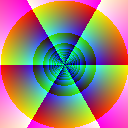What are in this table/image ?

The red channel: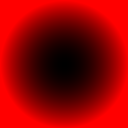Every byte in this channel describes how bright or dark a texel of a texture are rendered.

The green channel.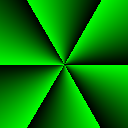Every byte in this channel describes which texel of a texture must be read based on the X direction.

The blue channel.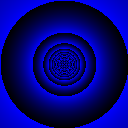Every byte in this channel describes which texel of a texture must be read based on the Y direction.

pseudo code:

Code: Select all

`loop   loop y all rows    loop x all cols      index = (x+y*rows+frame and mask)      plot x,y texture(table(index).green + table(index).blue*texturesize) * texture(index).red    end loop  end loop  frame+=1end loop`
The trick:
If you inc- or decrement the index that points in the table by a value (in this case number of frame)
the masking guaranteed always a legal location.

The result are the reading texels are changed this way so it looks like a animation.

Another point are the texture size is 256 x 256 pixels
in hex &HFFFF the high byte=y lo byte=x so it's easy to map any coords to a legal location.

Here are all together no magic ! ;-)

Joshy

Code: Select all

`'' main'dim as integer w,hscreeninfo ,h : h*=.8 : w=hscreenres w,h,32dim as integer TexturePitch,BufferPitch,TablePitchdim as ulong ptr pTexture,pBuffer,pTable' create images drawbuffer, tableimage and texture dim as any ptr imgBuffer =imagecreate(w,h,0)dim as any ptr imgTable  =imagecreate(w,h,0)dim as any ptr imgTexture=imagecreate(256,256,0)' get pixel pointers and pitch of the imagesimageinfo imgBuffer ,,,,BufferPitch ,pBufferimageinfo imgTable  ,,,,TablePitch  ,pTableimageinfo imgTexture,,,,TexturePitch,pTexture' convert bytes per row to pixels per rowTexturePitch shr=2BufferPitch  shr=2TablePitch   shr=2' put something in the 256 x 256 pixel texturedim as integer nfor y as integer = 0 to 256 step 64  for x as integer = 0 to 256 step 64    line imgTexture,(x,y)-step(65,65),RGB(x,y,x+y),BF    line imgTexture,(x,y)-step(65,65),RGB(255,255,255),B    n+=1  nextnextfor j as integer=0 to h-1  dim as single sy =  1 - j*(2/h)  for i as integer=0 to w-1    dim as single sx = -1 + i*(2/w)    dim as single sr = sqr(sx*sx+sy*sy)    dim as single sa = atan2(sy,sx)    dim as single su = 1/sr    dim as single sv = sa * 3/3.14159    dim as single sw = sr * sr    if ( sw>1 ) then sw=1    dim as long iu = su*255    dim as long iv = sv*255    dim as long iw = sw*255    pTable[j*TablePitch+i] = ((iw and &HFF) shl 16) or ((iv and &HFF) shl 8) or (iu and &HFF)  nextnextdim as single r,sdim as integer offsetwhile inkey()=""  s=sin(r)*20:r+=0.01  windowtitle "s:" & s  offset+=1+s  for j as integer = 0 to h-1    for i as integer = 0 to w-1      dim as const long ival = pTable[j*TablePitch+i]      dim as const long icol = pTexture[ ( (ival and &HFFFF) + offset) and &HFFFF ]      dim as const long isca = ival shr 16      pBuffer[j*BufferPitch+i] = ((((icol and &HFF00FF)*isca) shr 8) and &HFF00FF)  _                                + ((((icol and &H00FF00)*isca) shr 8) and &H00FF00)    next  next  put (0,0),imgBuffer,PSET   sleep 10wend`
Last edited by D.J.Peters on Sep 25, 2017 16:22, edited 2 times in total.
v1ctor
Posts: 3799
Joined: May 27, 2005 8:08
Location: SP / Bra[s]il
Contact:

### Re: Demo

Long time not see a tunnel effect :)

I took your last demo and compiled it to javascript using the work in progress Emscripten port.

Take a look: http://freebasic.net/temp/js-tunnel/tunnel.html

On the desktop (Windows 10), it runs great in Firefox and Edge, and OK in Chrome. IE 11 can also run it, but it's a bit slow.

I tested it too on my Android phone (Note 3). Runs fine in the stock browser, and slow in Chrome.
Posts: 2007
Joined: May 24, 2007 22:10
Location: The Netherlands

### Re: Demo

Tested the javascript version on my system, Xubuntu 14.04 64bit, with:
* Firefox: Smooth